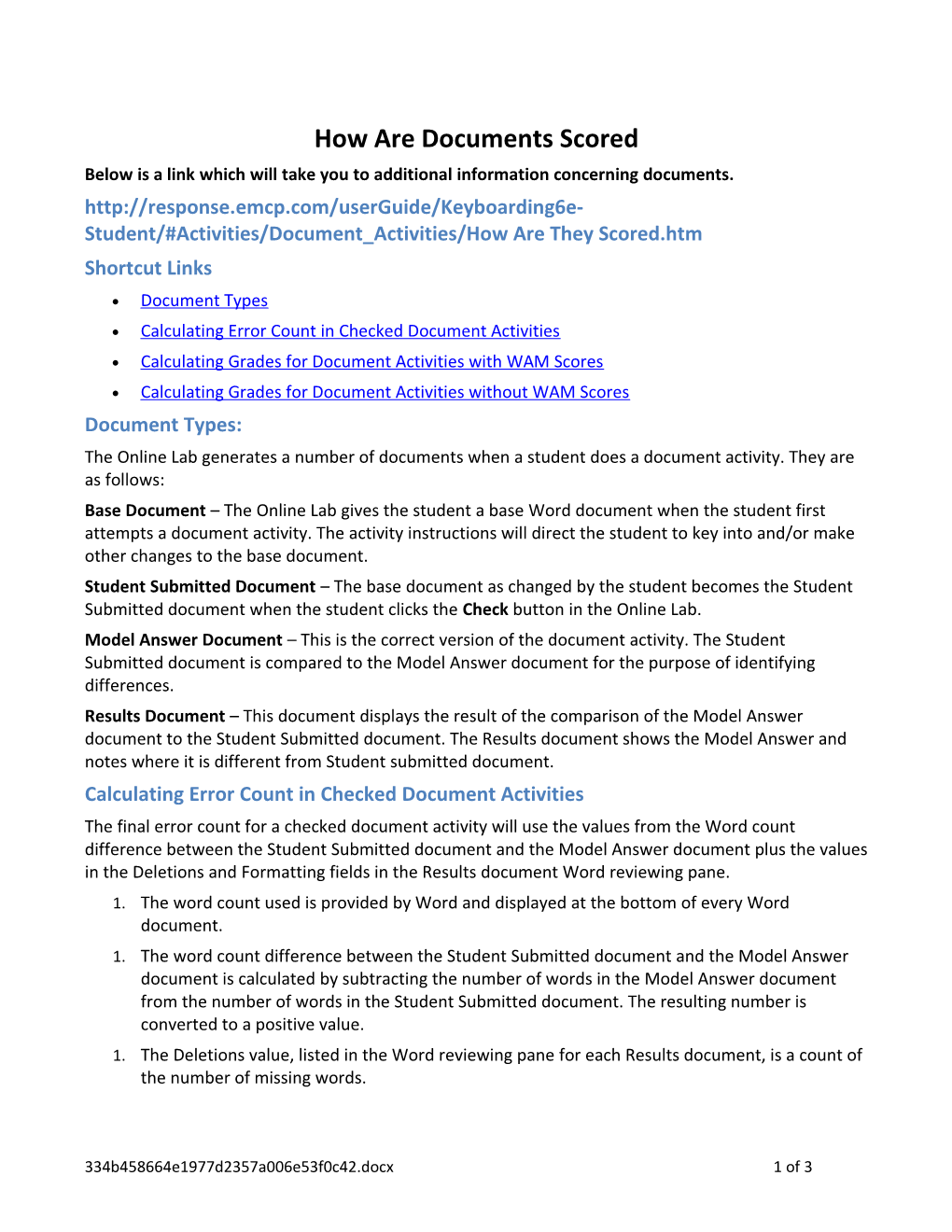# How Are Documents ScoredHow Are Documents Scored

Below is a link which will take you to additional information concerning documents.

Are They Scored.htm

• Document Types
• Calculating Error Count in Checked Document Activities
• Calculating Grades for Document Activities with WAM Scores
• Calculating Grades for Document Activities without WAM Scores

Document Types:

The Online Lab generates a number of documents when a student does a document activity. They are as follows:

Base Document – The Online Lab gives the student a base Word document when the student first attempts a document activity. The activity instructions will direct the student to key into and/or make other changes to the base document.

Student Submitted Document – The base document as changed by the student becomes the Student Submitted document when the student clicks the Check button in the Online Lab.

Model Answer Document – This is the correct version of the document activity. The Student Submitted document is compared to the Model Answer document for the purpose of identifying differences.

Results Document – This document displays the result of the comparison of the Model Answer document to the Student Submitted document. The Results document shows the Model Answer and notes where it is different from Student submitted document.

Calculating Error Count in Checked Document Activities

The final error count for a checked document activity will use the values from the Word count difference between the Student Submitted document and the Model Answer document plus the values in the Deletions and Formatting fields in the Results document Word reviewing pane.

1. The word count used is provided by Word and displayed at the bottom of every Word document.
1. The word count difference between the Student Submitted document and the Model Answer document is calculated by subtracting the number of words in the Model Answer document from the number of words in the Student Submitted document. The resulting number is converted to a positive value.
1. The Deletions value, listed in the Word reviewing pane for each Results document, is a count of the number of missing words.
1. The Formatting value, listed in the Word reviewing pane for each Results document, is the actual count of the format differences between the submitted document and the model answer document.
1. The error count is the sum of the following: word count difference + Deletions + Formatting.

Calculating Grades for Document Activities with WAM Scores

Grading Method – Method used by the LMS to determine activity grading method in the grade book

Max Errors Allowed – Total errors allowed in the graded activity. If the student’s work has more errors than Max Errors Allowed, then the grade is an F. If the student’s work has less than or equal to the errors of Max Errors Allowed, then the grade is based on the WAM value.

WAM Value – The WAM Value is the minimum number of words-per-minute the student needs to achieve to receive a grade of an A. A reduction in 5 strokes from the WAM Value is the words-per-minute the student needs to achieve to receive a grade of a B, and so on for C, D, E, and F. The WAM Value could be different from one activity to another activity. However, in all instances, the WAM Value (in the table) represents the words-per-minute the student needs to achieve to receive a grade of an A.

If the count of errors in the activity of a student’s work is less than or equal to Max Errors Allowed:

Grade Value = A, B, C, D, or F. Each grade is a 5 stroke reduction of the previous WAM Value starting with the A value. If a student’s WAM reaches the WAM Value, the grade is an A. If the student’s WAM rate is 5 strokes less that the WAM rate Value, then the grade is a B, and so on.

Student WAM >=30, Grade is A,
Student WAM >=25 and <30, Grade is B,
Student WAM>=20 and <25, Grade is C,
Student WAM>=15 and<20, Grade is D,

If the number of errors in a student's work is greater than Max Errors Allowed: Grade value is F.

Calculating Grades for Document Activities without WAM Scores

Max Errors – Total errors allowed in the graded activity Checked Document Activity - Focus on Formatting. If the student’s work has more errors than Max Errors, then the grade is a zero. If the student’s work has less than or equal to the errors of Max Errors, then the grade value formula is used.

Error Count – The actual number of errors in the student document using the method described in the above section Calculating Error Count in Checked Documents Activities.

If the count of errors in the activity of a student’s work is less than or equal to Max Errors Allowed:

Grade value = percent correct. Is computed using the following formula, where ME is Max Error and EC is Error Count.